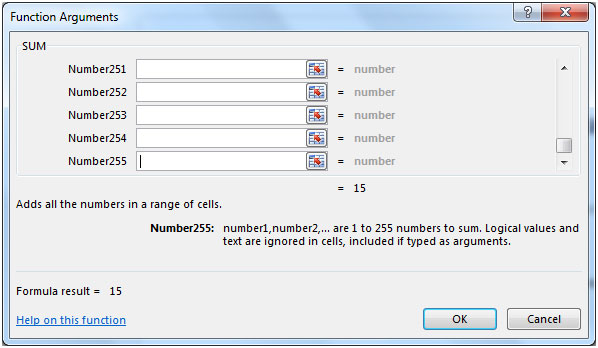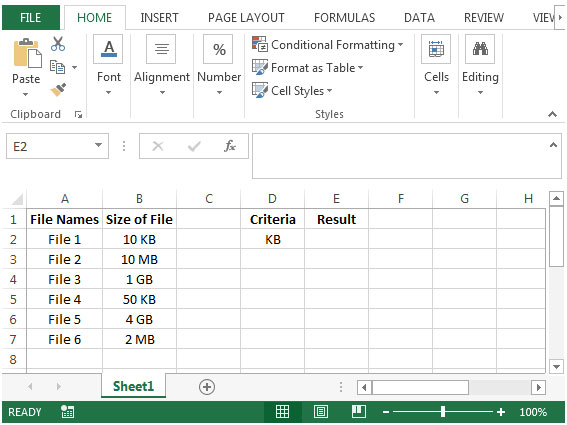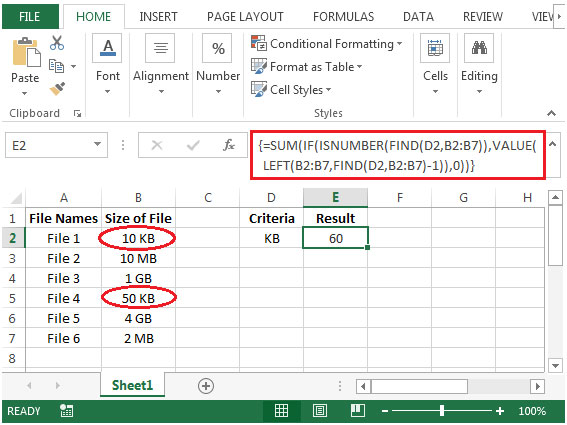How to Sum Values based on Numerical & Textual Substring in Microsoft Excel 2010

To find the sum of values from a list containing numerical & textual excel substring, we will use a combination of "SUM", "IF", "ISNUMBER", "LEFT", "FIND" & "VALUE" functions to retrieve the output.
SUM: Adds all the numbers in a range of cells

Syntax: =SUM(number1,number2,...)

There can be maximum 255 arguments. Refer below shown screenshot:The IF function checks if a condition you specify is TRUE or FALSE. If the condition is TRUE then it returns preset value, and if the condition is FALSE then it returns another preset value.

Syntax = IF(logical_test,value_if_true,value_if_false)
ISNUMBER: Checks whether a value is a number, and returns TRUE or FALSE.

Syntax =ISNUMBER(value)
LEFT: Returns the specified number of characters from the start of a text string.

Syntax: =LEFT(text,num_chars)

FIND: Returns the starting position of one text string within another text string. FIND is a case sensitive function.

Syntax: =FIND(find_text,within_text,start_num)
VALUE: Converts a text string that represents a number to a number.

Syntax: =VALUE(text)
Let us take an example:

We have file names in column A & their size in column B.We need a formula to find the sum of totals for each type of file size based on the criteria set in cell D2. Each file size is one of three types (KB, MB or GB).• In cell E2, the formula would be
• {=SUM(IF(ISNUMBER(FIND(D2,B2:B7)),VALUE(LEFT(B2:B7,FIND(D2,B2:B7)-1)),0))}
• This is an array formula which requires formula to be enclosed with curly brackets by using CTRL + SHIFT + ENTER.The function will return the total value from the list which is containing numerical and textual substrings.

1.